Jet Set Go! All about Aeroplanes Jet Set Go! All about Aeroplanes

Addition is one of the four basic arithmetic operations in mathematics namely addition, subtraction, multiplication and division. This operator is used to add two or more numbers or things together. This plays a vital role in our daily existence while dealing with different types of transactions. Also, addition is the basic operation that is introduced to the students at their primary level. In this article, you are going to learn the meaning of addition, properties, addition on the number line, different addition techniques and formulas.

The four basic arithmetic operations are illustrated below: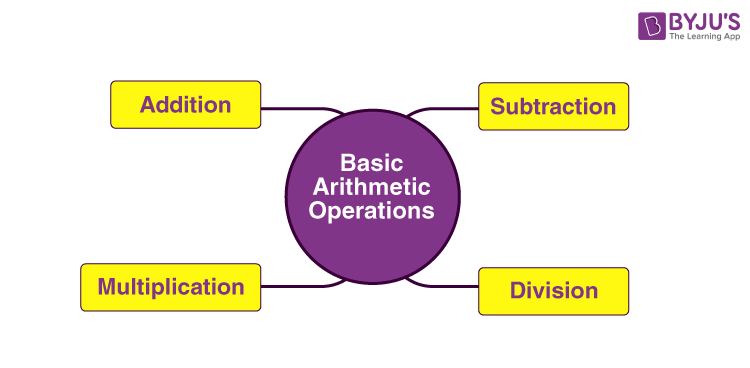The addition is the term used to describe adding two or more numbers together. The addition is denoted using the plus sign ‘+‘ such as the addition of 3 and 3 can be written as 3 + 3. Also, the plus sign (+) can be used as many times as required, such as 3 + 3 + 3 + 3.

Mathematical addition is an important aspect of the curriculum for children’s learning. Simple addition sums, such as one-digit facts, Maths addition sums, and double-digit Maths addition sums are taught in primary school. Addition sums are one of the most basic and engaging Math topics for primary school students. Math is a fascinating subject, and children acquire mathematical principles such as adding sums for the first time during their early years. The process of adding one or more integers to get a new total is one of the four basic operations of Arithmetic. In primary school, children are taught how to solve fundamental addition problems.

For lists of large numbers, it usually is more comfortable to write the list of numbers in a column and execute the calculation at the bottom. In this case, the addition of the list of numbers is termed as the sum and is represented using the symbol ∑.

The symbol used for addition is “+”. Whenever we perform significantly large numbers or more items, then we can use the sigma symbol (∑).

The parts of addition are shown in the below figure: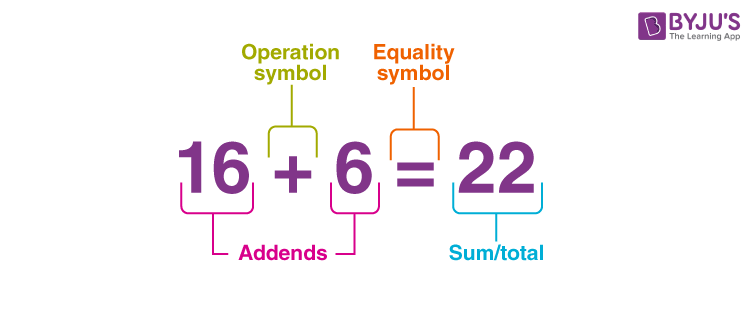The below figure shows the addition of simple numbers that helps in creating the individual addition tables of numbers from 1 to 10.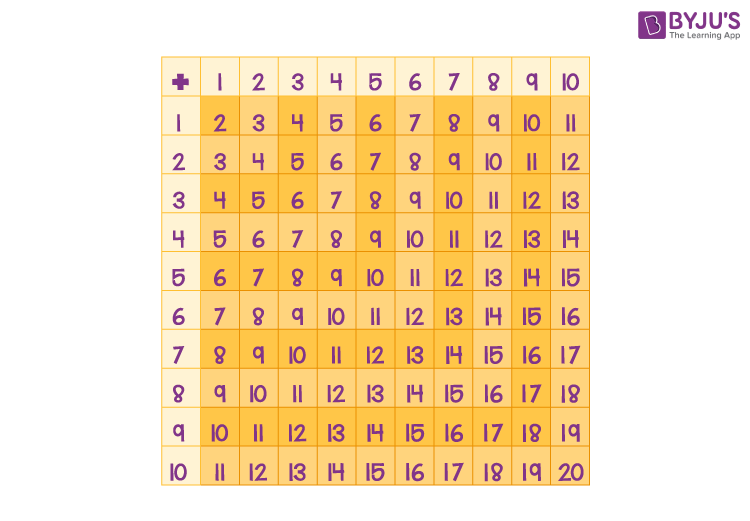The addition of two or more numbers possesses some important properties. The list of properties of addition given below will help in predicting the sign of the result obtained in the addition process.

• The addition of two whole numbers is again a whole number.
• The addition of two natural numbers will result in the natural number again.
• The addition of two integers will be an integer; the addition of two positive integers will be a positive integer, the addition of two negative integers will be a negative number and the addition of one positive and one negative integer will be an integer with the same sign as the sign of the largest number.
• The addition of two rational numbers will be a rational number again. Also, the property related to positive and negative signs will be the same as for integers.
• The addition of any number with 0 gives the number itself.

There are various strategies and methods to add numbers in maths and they are:

• Using counting figures (using fingers)
• Using the number line
• With regrouping

In the figure given below, 3 yellow balls and 6 purple balls are given. By counting the number of each coloured ball, we can say that there are a total of 9 balls.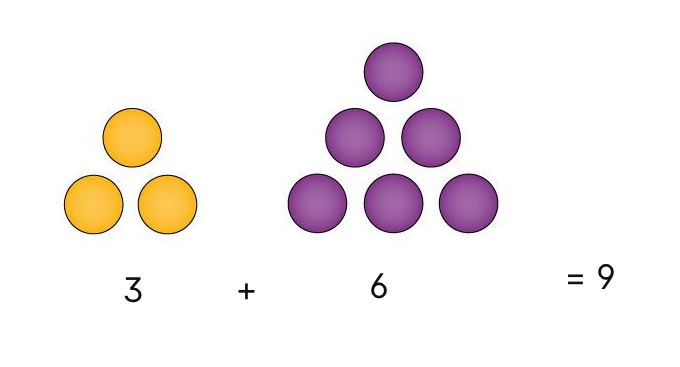The basic method of adding integers involves the representation of numbers on the number line and then adding them by counting the numbers. This can be understood clearly with the help of the below given example:

Example: Perform -3 + 5 on the number line.

Solution:

Given, -3 + 5

That means, five points to be added to -3.

This can be done using the number line as shown below: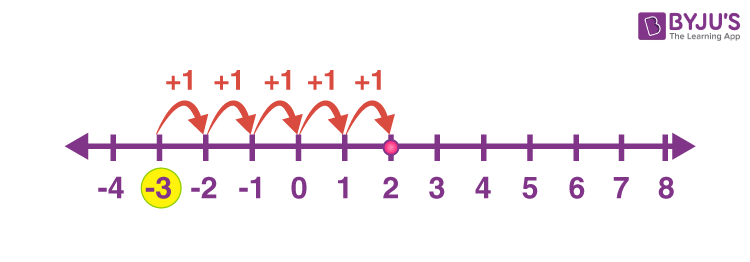Since the operation is in addition, we need to move towards the right of -3. The step towards the right of -3 to 5 units (as shown with red arrow curves). The final unitary addition ends at 2.

Therefore, -3 + 5 = -3 + 1 + 1 + 1 + 1 +1 = 2

As explained above, the addition of single digit numbers can be done easily on the number line. Now, the question comes to mind: how to add numbers with two or more digits? This can be done using regrouping of digits of the same place value together. In maths, regrouping can be defined as the process of creating groups of tens, hundreds, or thousands when carrying out some operations like addition and subtraction with two or more digit numbers.

Let’s have a look at the example given below:

Example: Add two numbers 57 and 46.

Solution:

57 + 46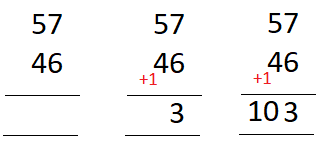When we add the digits of numbers from the bottom of the one’s column to the one on top, we get 7 + 6, which is equal to 13.

As we know, a maximum of 9 can be written in the one’s column so we can’t put the whole eleven there. We can write the digit representing the ones (i.e. 3) in the one’s column, and the other digit (i.e. digit 1 from 13) gets regrouped to the tens column digits.

Now, we can complete this by adding the numbers in the tens column: 5 + 4.

Do not forget to add the regrouped ten as well, i.e. 5 + 4 + 1.

This equals 10 so write it in the tens column, as a total of 103.

A similar approach can be used to add three or more digit numbers.

Besides, we have different approaches to add the given numbers apart from the number line and grouping techniques. Thus, we can also add numbers by counting fingers (for smaller numbers).

Also, get addition worksheets here for more practice.

Question 1: In a little champs musical show, 1201 girls and 1389 boys participated. What is the total number of participants?

Solution:

Number of girl participants = 1201

Number of boys who participated in the show = 1389

Total number of participants = 1201 + 1389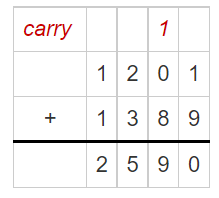Hence, the total number of participants = 2590.

Question 2: In a school, there are 125 students in section A, 164 students in section B and 147 students in section C of class X. Find the total number of students of class X if there are only three sections for this class.

Solution:

Number of students in section A = 125

Number of students in section B = 164

Number of students in section C = 147

Total number of students = 125 + 164 + 147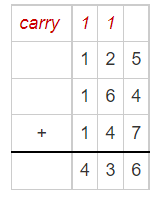Therefore, the total number of students in class X = 436

### Practice Problems

1. Add 18, 42 and 23.
2. Find the sum of 110 and 236.
3. Neha has a garden with 13 mango trees, 4 papaya trees and 6 banana trees. How many trees are there in her garden?
4. Mayank ate 4 chocolates on Sunday, 3 on Monday, 2 on Tuesday, 5 on Wednesday, 4 on Thursday, 5 on Friday and 6 on Saturday. How many chocolates did he ate in the week?

### What is the meaning of addition?

Addition is one of the four basic arithmetic operations. The addition is the act of combining two or more things, numbers, objects, and so on. The symbol used for addition is +.

### What are the parts of Addition?

There are 3 main parts of addition, namely the addend, the equal sign, and the sum.

### How many types of addition strategies are there?

There are different strategies to perform addition such as:
Using a number line